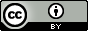hypothesis testingBasic Statistics

•• Category : Natural Sciences > Math
• Taught by : Phoutsakhone Channgakham
• Created By : National University of Laos
• Offered By : ASEAN Cyber University
• Hits : 270
• Keyword : steps in hypothesis testing, z-test for mean, t-test for mean, z-test for proportion, X2-test for variance or standard deviation

"This charpter explain about steps in hypothesis testing, z-test for mean, t-test for mean,z-test for proportion, X2-test for variance or standard deviation 1. steps in hypothesis testing, z-test for mean - This material explains about steps of hypothesis testing and test hypothesis by Z-test for mean 2. t-test for mean - This material explains about steps of hypothesis testing and test hypothesis by T-test for mean 3. z-test for proportion - This material explains about steps of hypothesis testing and test hypothesis by T-test for proportion 4. X2-test for variance or standard deviation - This material explains about steps of hypothesis testing and test hypothesis by X2-test for variance or standard deviation"